# 4-4 Fitts Law and Hick s Law

 Team-Fly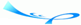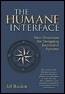Human Interface, The: New Directions for Designing Interactive SystemsBy Jef Raskin Table of Contents Chapter Four.  Quantification

### 4-4 Fitts' Law and Hick's Law

It behooves us to place the foundations of knowledge in mathematics.

?span>Roger Bacon, Opus Majus (13th century)

Various quantitative laws relating to interface design have sound cognetic underpinnings and have been validated repeatedly. These laws often give you additional data on which you can base interface-design decisions. Fitts' law quantifies the fact that the farther a target is from your current cursor position or the smaller the target is, the longer it will take you to move the cursor to the target. Hick's law quantifies the observation that the more choices of a given kind you have, the longer it takes you to come to a decision.

#### 4-4-1 Fitts' Law

Consider that you are moving a cursor toward an on-screen button. The button is the target of the move. The length of a straight line from the position at which the cursor started to the closest point on the target is the distance used in the statement of Fitts' law. Given the size of the target and the distance to be moved, Fitts' law gives you the average time it takes a user to succeed in getting the cursor to the button.

In the one-dimensional case, in which the target's size, measured along the line of motion, is S and the target is at a distance D from the starting position (Figure 4.6), Fitts' law states that

Time (in msec) = a + b log2 (D / S + 1)

##### Figure 4.6. Distances used in Fitts' law to determine the time to move a cursor to a target.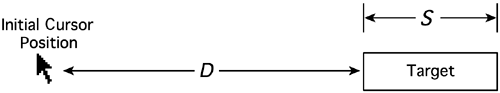(The constants a and b are determined experimentally or are derived from human performance parameters.) The time that you calculate begins when the cursor is at the starting point and after the user has chosen the target. The logarithm to the base 2 gives a measure of the difficulty of the task in terms of the number of bits of information it takes to describe the (one-dimensional) path of the cursor.

 Mathematics, supposedly a paragon of clarity, clings yet to that old-fashioned style whereby undefined variables appears in a formula before you know what they stand for. For example, you will see such statements as

A = p r2,

where r is the radius of a circle and A is its area.

This can be confusing, forcing you to read ahead and then go back, especially if the equation is a long one with lots of as-yet-unexplained variables. Far better, from a reader's viewpoint, is to follow the obvious dictum to define terms before you use them:

A circle with radius r has an area A, given by:

A = p r2

The units of distance do not affect the calculated time, because D / S is the ratio of two distances and is therefore dimensionless. It follows that, even though we might move the pointing device a distance smaller or larger than the distance the cursor moves on the display, the law still works when the distances are measured on the display, assuming a linear relationship between GID and cursor motion. Fitts' law applies only to the kinds of motions we make when we are using most human-machine interfaces: motions that are small relative to human body size and that are uninterrupted, that is, movements that can be made in one continuous motion. For back-of-the-envelope approximations, I use a = 50 and b = 150 in the Fitts' law equation.

An extension of Fitts' law to more complex constraints, such as tracking a cursor between straight or curved walls, has been developed and tested empirically (Accot and Zhai 1997). For a two-dimensional target, you can usually obtain a reasonable approximation of the time needed to move the cursor to the target, using the smaller of the horizontal and vertical dimensions of the target for the value of S (Mackenzie 1995).

Fitts' law explains, for example, why it is much faster to move the cursor to an Apple Macintosh style menu (Figure 4.7) that is on the edge of a display than to a Microsoft Windows style menu (Figure 4.8) that floats away from an edge. The size S of the Windows menu on my display is 5 mm. The effective size of the Macintosh target is large because you do not have to stop within the confines of the menu bar but rather can continue to move the GID any comfortable distance beyond that needed to put the cursor in the menu: The cursor stops at the edge of the display.

##### Figure 4.7. The Macintosh menu, at the top edge of the screen, effectively increases its size compared to a menu that floats away from the edge.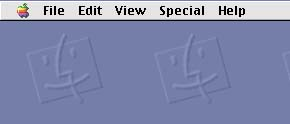##### Figure 4.8. The Windows menu is below the top edge of the screen; you have to place the cursor more carefully to pull down a submenu.A series of tests I performed determined that users typically stop within about 50 mm of the edge of the display on the Macintosh, so we can use 50 mm as S for the Macintosh. On a 14-inch flat panel display, the average distance the cursor must be moved to reach the menu bars is 80 mm; thus, the calculated time to move the cursor to a menu item on the Macintosh is

50 + 150 log2 (80 / 50 + 1) = 256 msec.

This result is far less than the calculated time it takes to move the cursor to a corresponding menu item on a Windows-style menu:

50 + 150 log2 (80 / 5 + 1) = 663 msec.

These calculations apply only to the time it takes the user to move the cursor. Clicking on the target to indicate that you believe that the cursor has reached its goal adds another 0.1 sec on average. (K = 0.2 in the GOMS model includes both the downstroke and the release of the button, whereas this timing is stopped by the downstroke.) In a typical experimental situation, you have to add the human reaction time of about 0.25 sec at the start of the cursor movement. When we take these factors into account, we get times that agree with what I have observed: It takes about 0.6 sec, on average, for a user to open an Apple menu, whereas it takes a user more than 1 sec to open a Windows menu. This analysis makes it clear why menus were deliberately placed at the edge of the display when the Macintosh interface was developed.

#### 4-4-2 Hick's Law

Before you move the cursor to a target or take any one action with a multiplicity of choices, you must first choose the target or action. Hick's law says that when you have to choose to take one among n alternative actions and when the probabilities of taking each alternative are equal, the time to choose one of them is proportional to the logarithm to the base 2 of the number of choices plus 1. When put this way, Hick's law looks just like Fitts' law:

Equation 1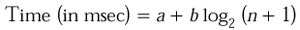If the probability of the ith choice is p(i), then, instead of the logarithmic factor in the equation, you use

Equation 2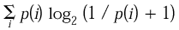The coefficients in Hick's law are strongly dependent on many conditions, including how the choices are presented and how habituated to the system the user has become. (If the choices are presented in a confusing manner, both a and b can increase; habituation decreases b.) These dependencies will not be discussed here; all we need to consider is that making decisions takes time, that making complex decisions takes more time than making simple ones, and that the relationship is logarithmic. In the absence of better information, we can use the same coefficients a and b as for Fitts' law to make off-the-cuff or relative estimates.

Whatever positive, nonzero coefficients we use for a and b, it follows from Hick's law that giving a user many choices simultaneously is usually faster than is organizing the same choices into hierarchical groups. Making choices from one menu of eight items is faster than is making choices from two menus of four items each. Assuming that the items are equally likely to be chosen and ignoring the time it takes to open the second menu, which, if taken into account, would make the time taken for the two-menu interface even longer we compare the time to select one item of eight, a + b log2 8, with the time to select one item of four twice, 2 (a + b log2 4). Because log2 9 = 3 and log2 5 = 2, and because both a < 2a and 3b < 4b, we see that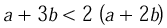This accords with experiments on menu structures (see, for example, Norman and Chin 1988).

Our discussion of Fitts' and Hick's laws is incomplete. For example, it is no accident that they have the same form as the Shannon-Hartley theorem. Nonetheless, this brief treatment is sufficient to alert you to these useful guides to interface design. They can help you even if, as in the example, you do not know the empirical coefficients a and b. (For more detail, see Card, Moran, and Newell 1983, pp. 72?4.)

Another instance of the tight relation between interface design, infor mation theory, and thermodynamics is apparent when you note that the equation (2) on page 96 is also the equation for the entropy of the probability distribution p (see Bennett 1981).

 Team-FlyTopThe Humane Interface. New Directions for Designing Interactive Systems ACM Press Series
ISBN: 1591403723
EAN: N/A
Year: 2000
Pages: 54